# NCERT Solutions for Class 10 Maths Chapter 11 Constructions

NCERT Solutions for Class 10 Maths Chapter 11 Constructions

This chapter contains the solutions to two exercises of chapter 11 constructions. Both the practiced exercises include answering questions related to Chapter 11, i.e., Constructions. In this chapter, students are given the solutions to many questions to perform well in their first term examinations.

The solutions to the NCERT-based questions of Chapter 11 for Class 10 Maths, Constructions, is an essential study resource for Class 10 students. These assist students in comprehending or solving the sorts of problems that a paper setter asked 2023 examinations of CBSE Class 10 Maths. Furthermore, offering solutions to all areas relating to circles assists students in effectively preparing for the Term I examinations.

The subject matter experts make sure that the errors in the NCERT textbooks are resolved according to the NCERT rules and syllabus.

NCERT Solutions for Class 10 Maths Chapter 11 Constructions | Free PDF Download

The solutions to the NCERT-based questions of Chapter 11 Constructions for class 10 Maths are accessible at our website. These solutions will assist students in acing their 2023 examinations by preparing for the examinations ahead of time. Students may clarify all of their conceptual questions or doubts with the assistance of the notes of Maths NCERT Solutions for Class 10 of this chapter which we provide. Experts have created these NCERT Solutions based on the most recent update to the CBSE syllabus for 2022-23 to help students in Class 10 prepare well for their 2023 examinations.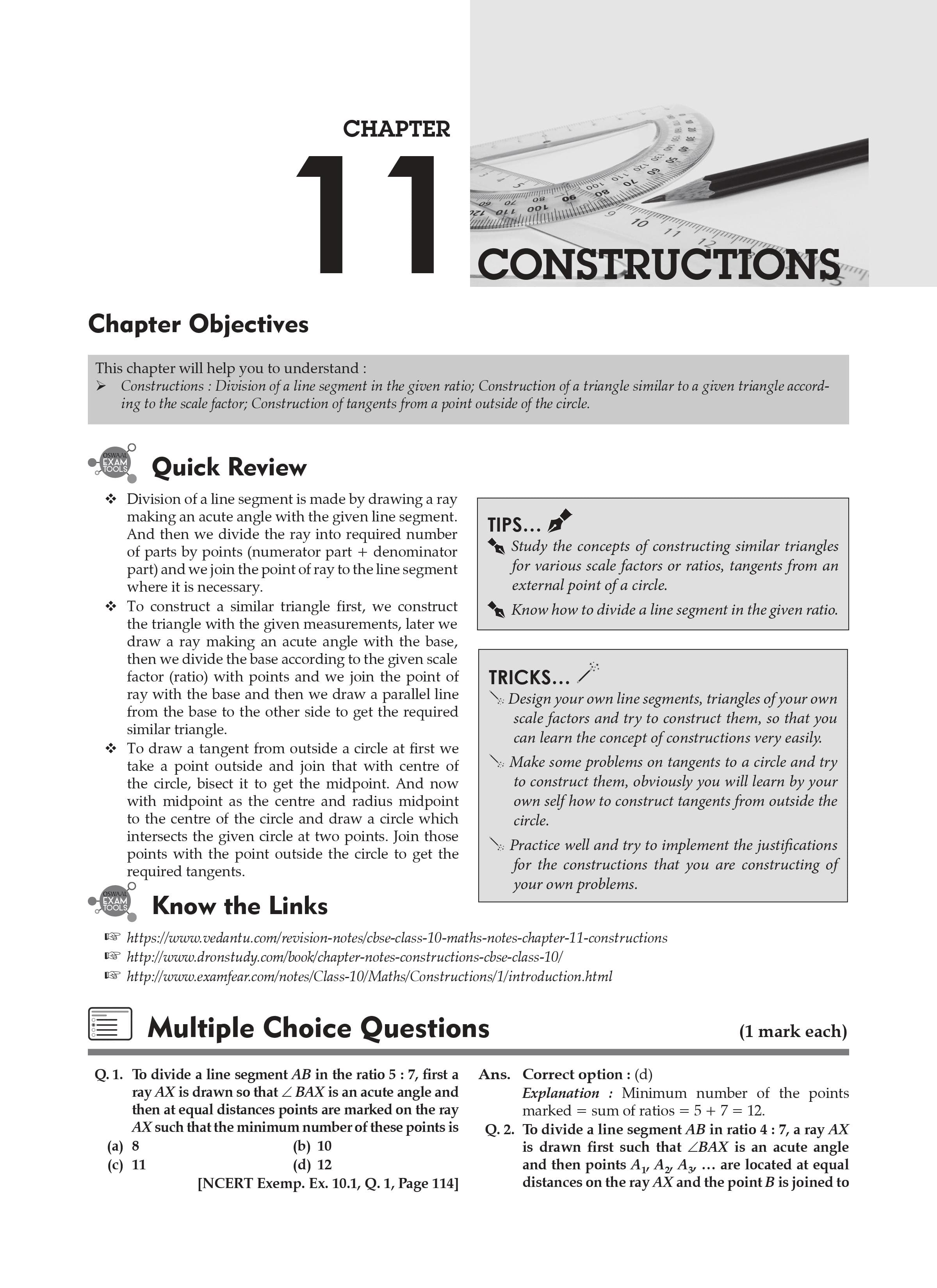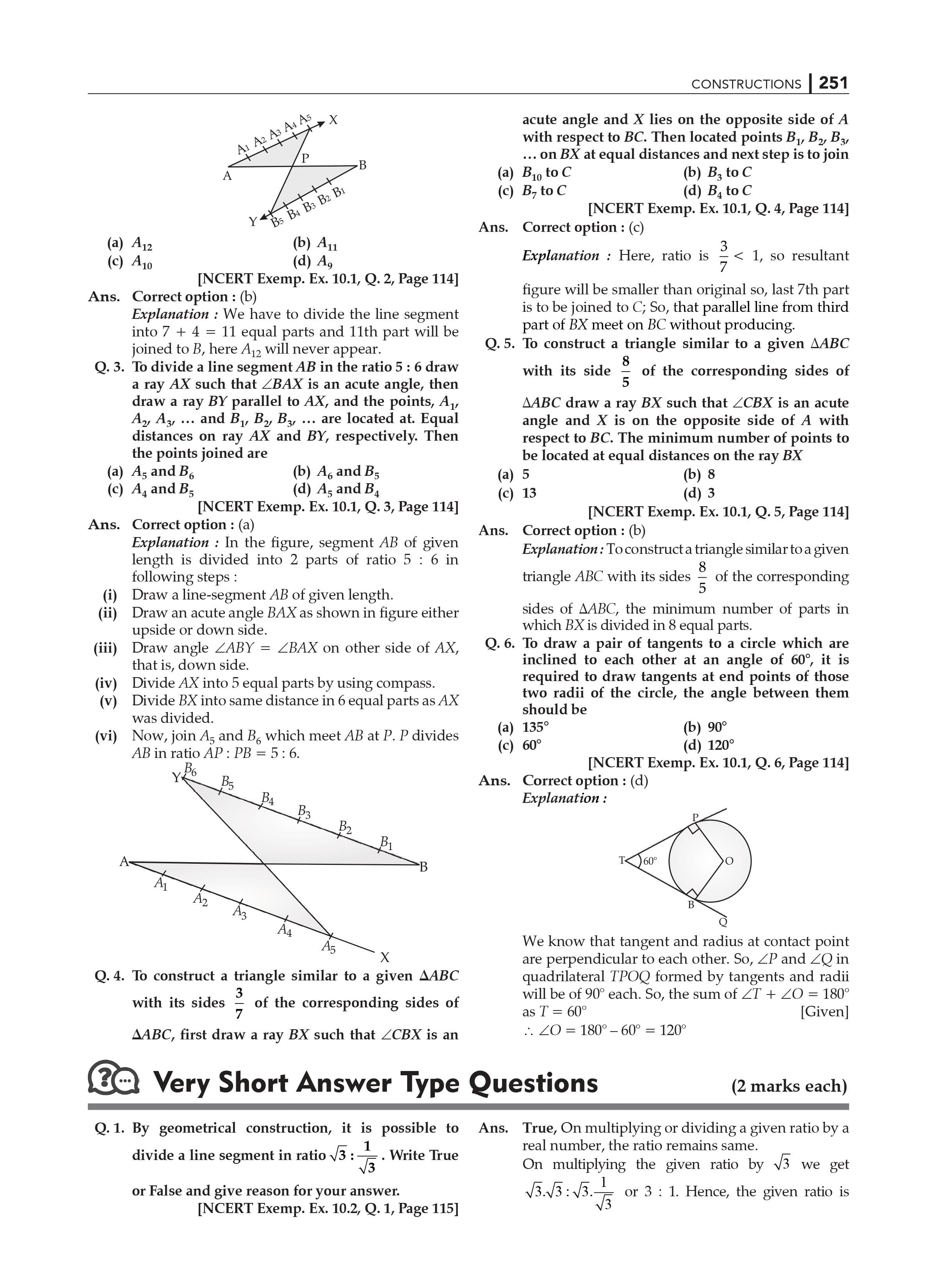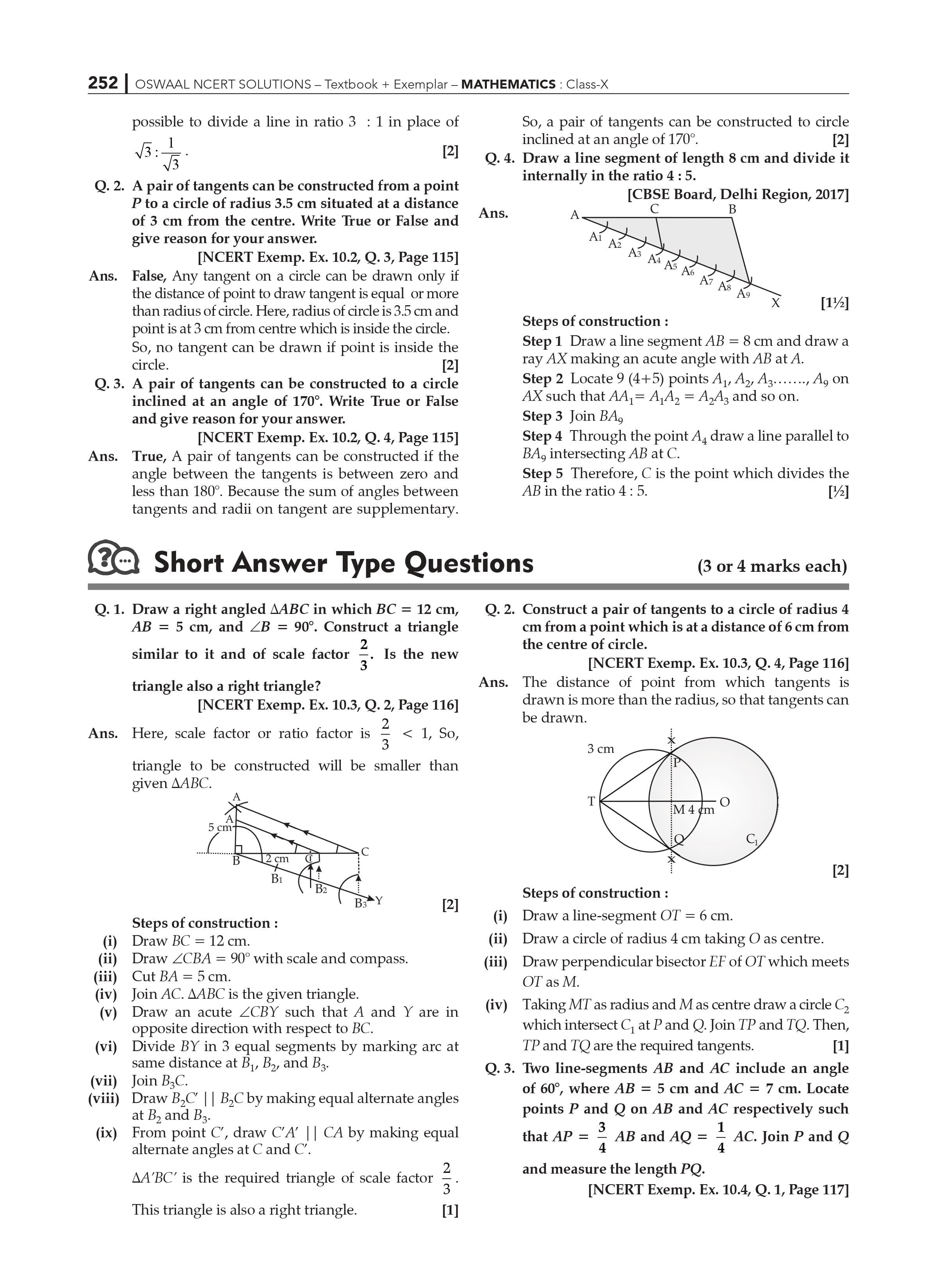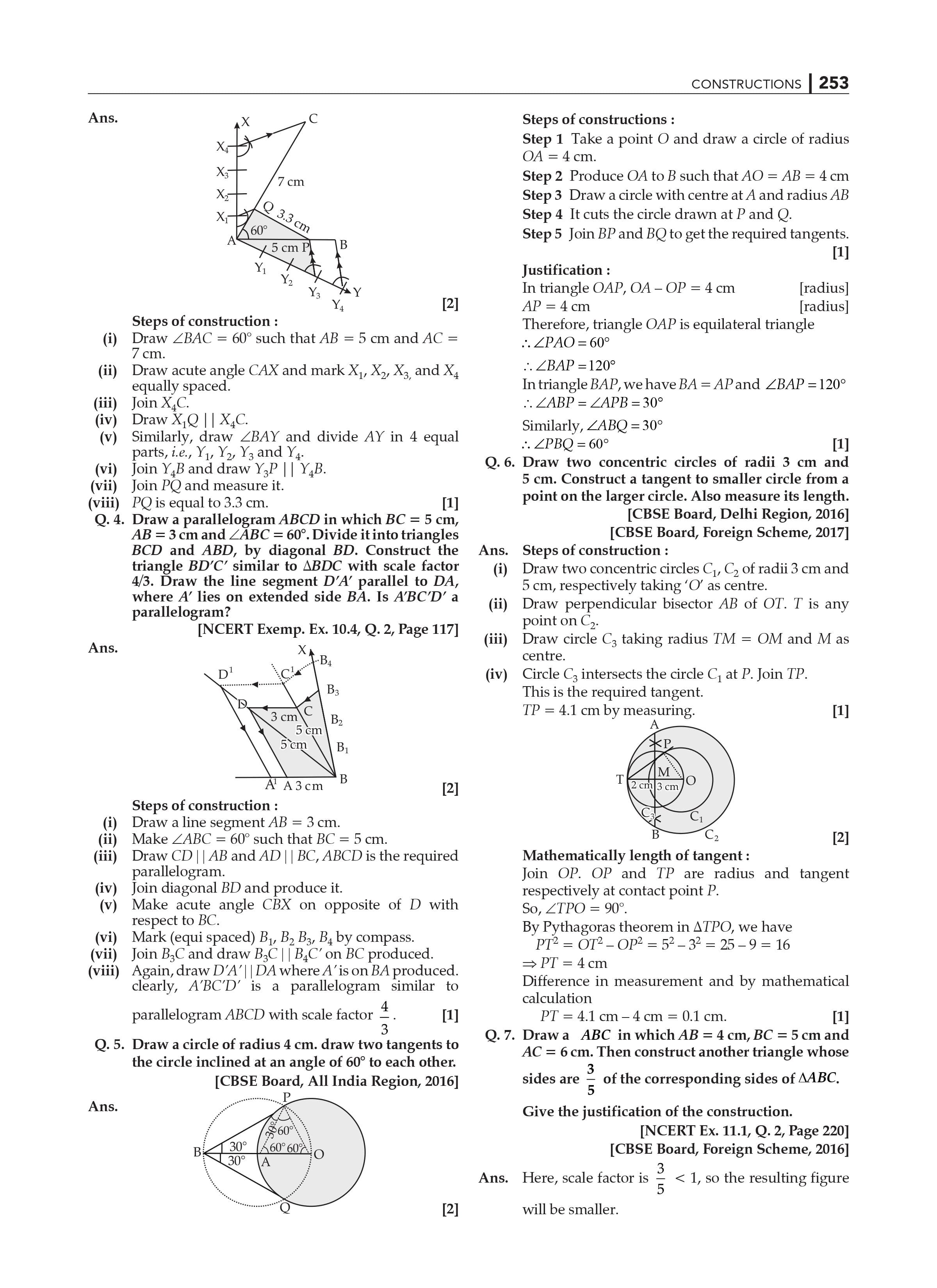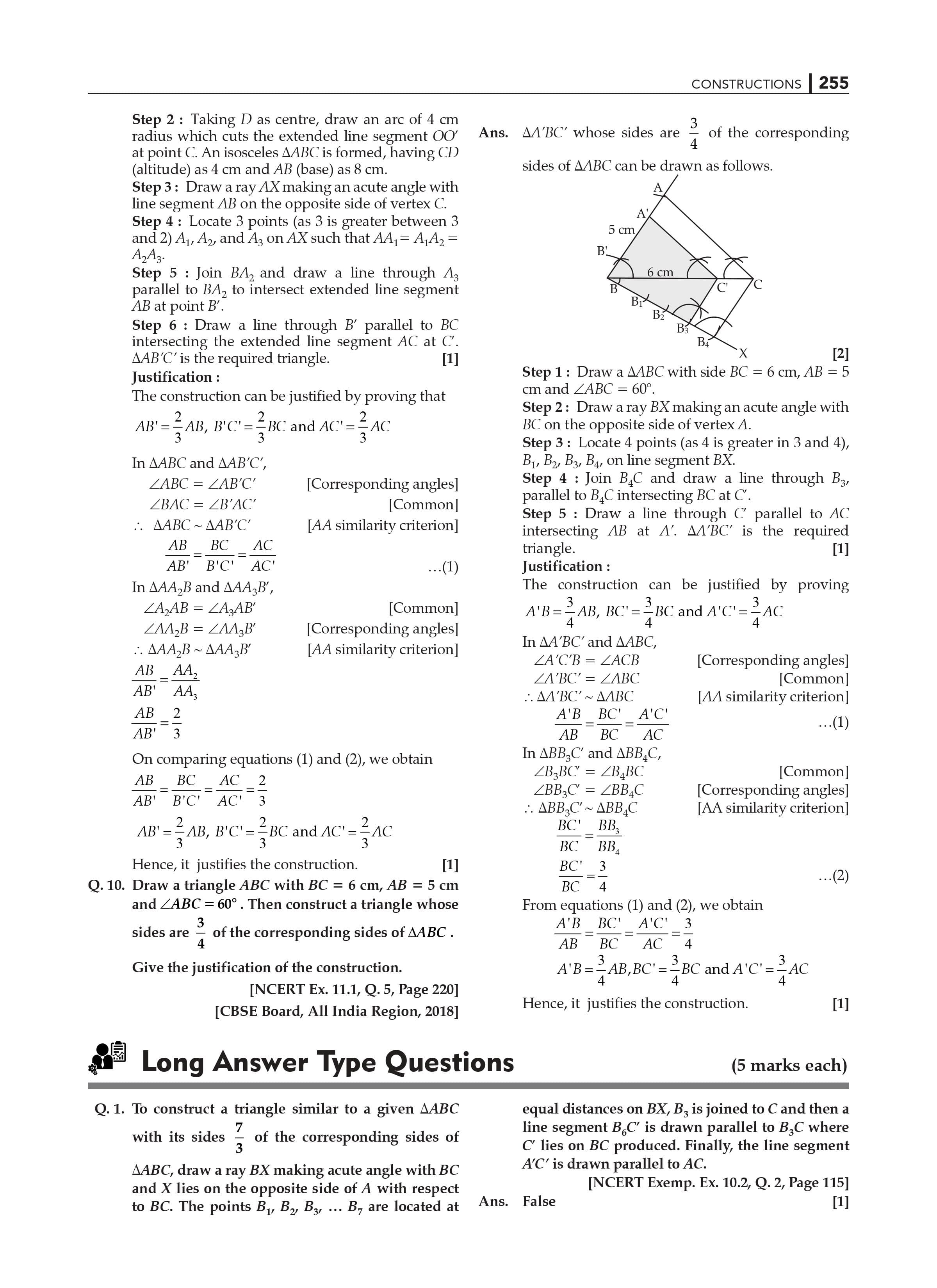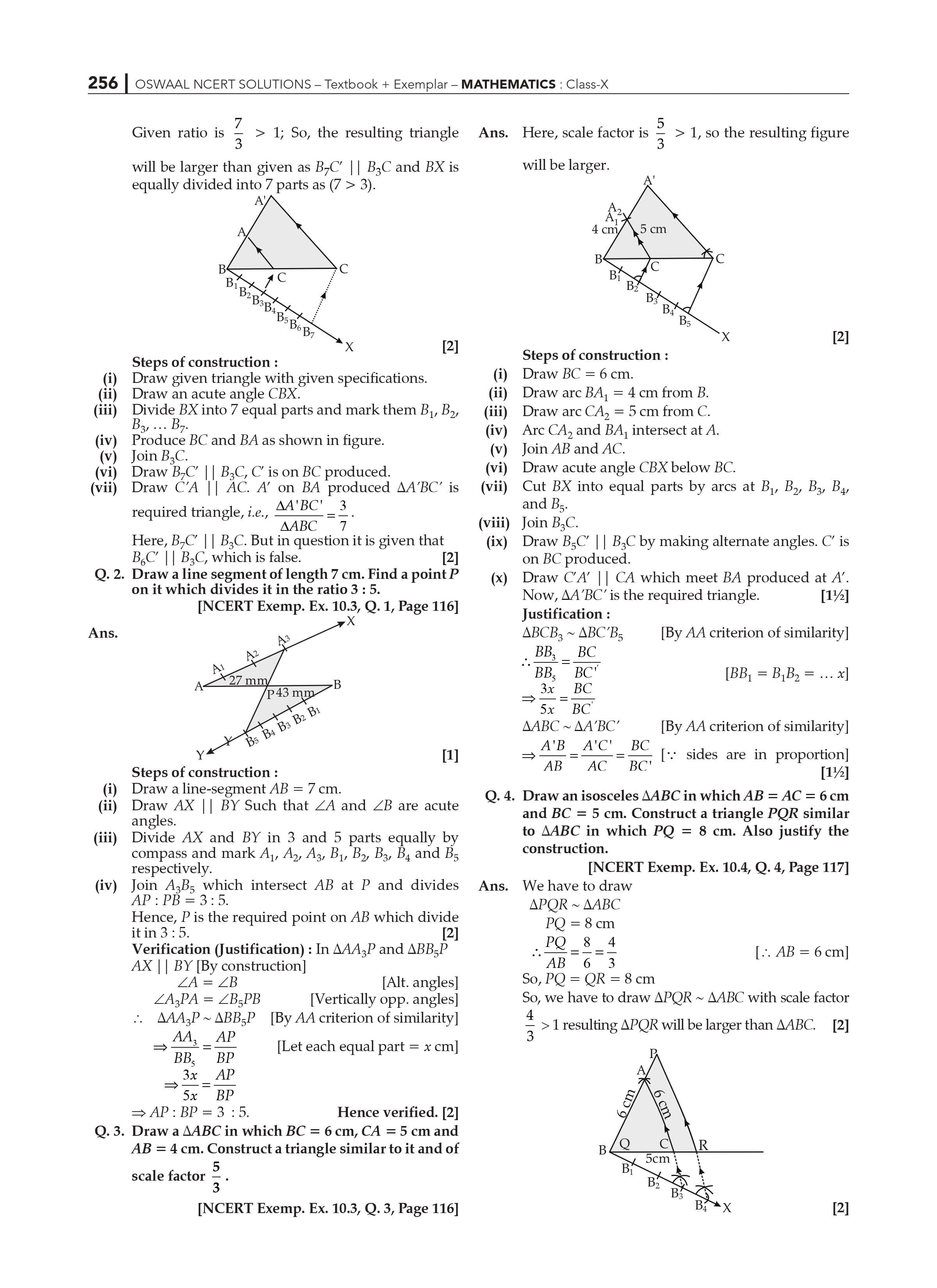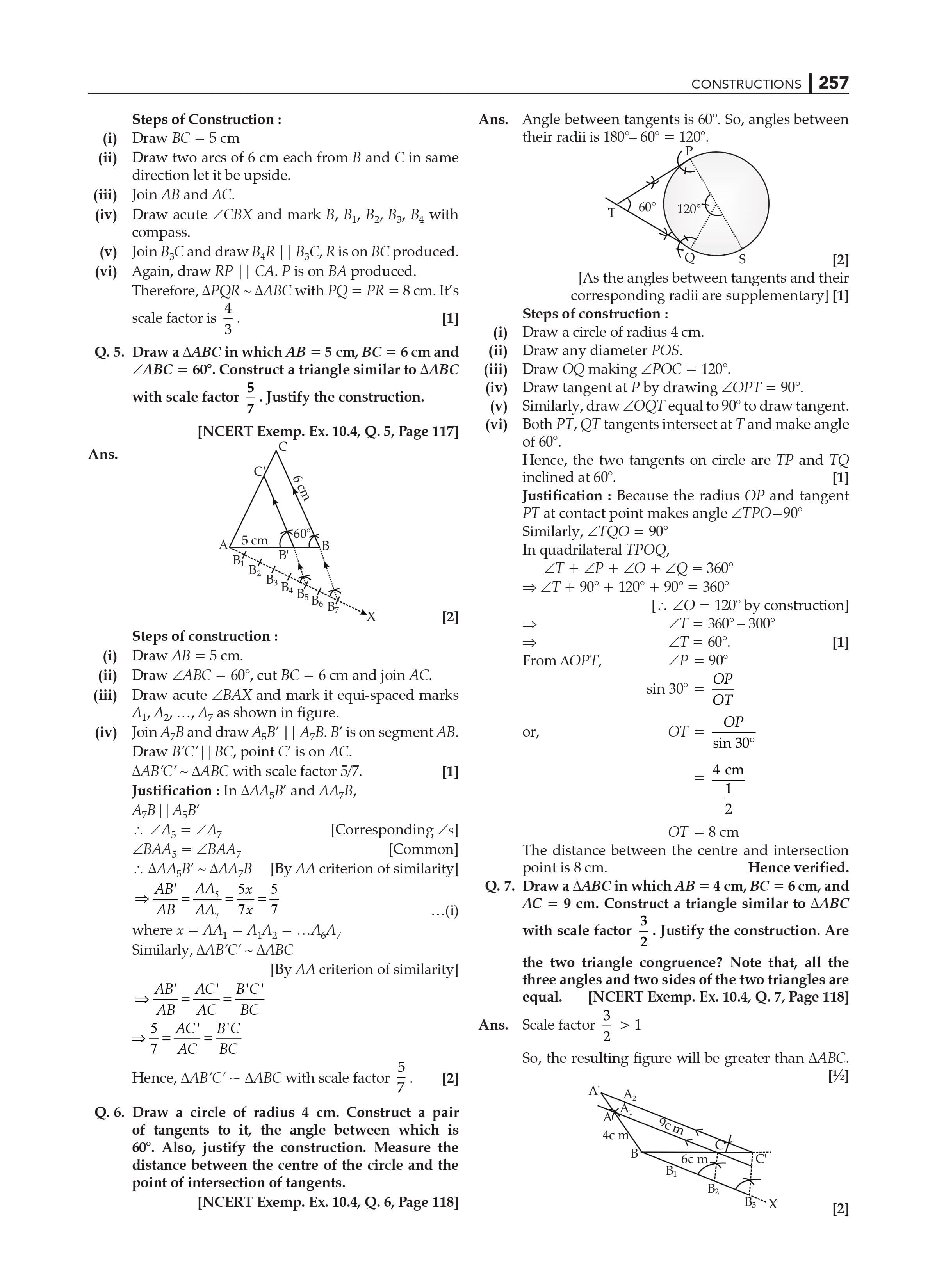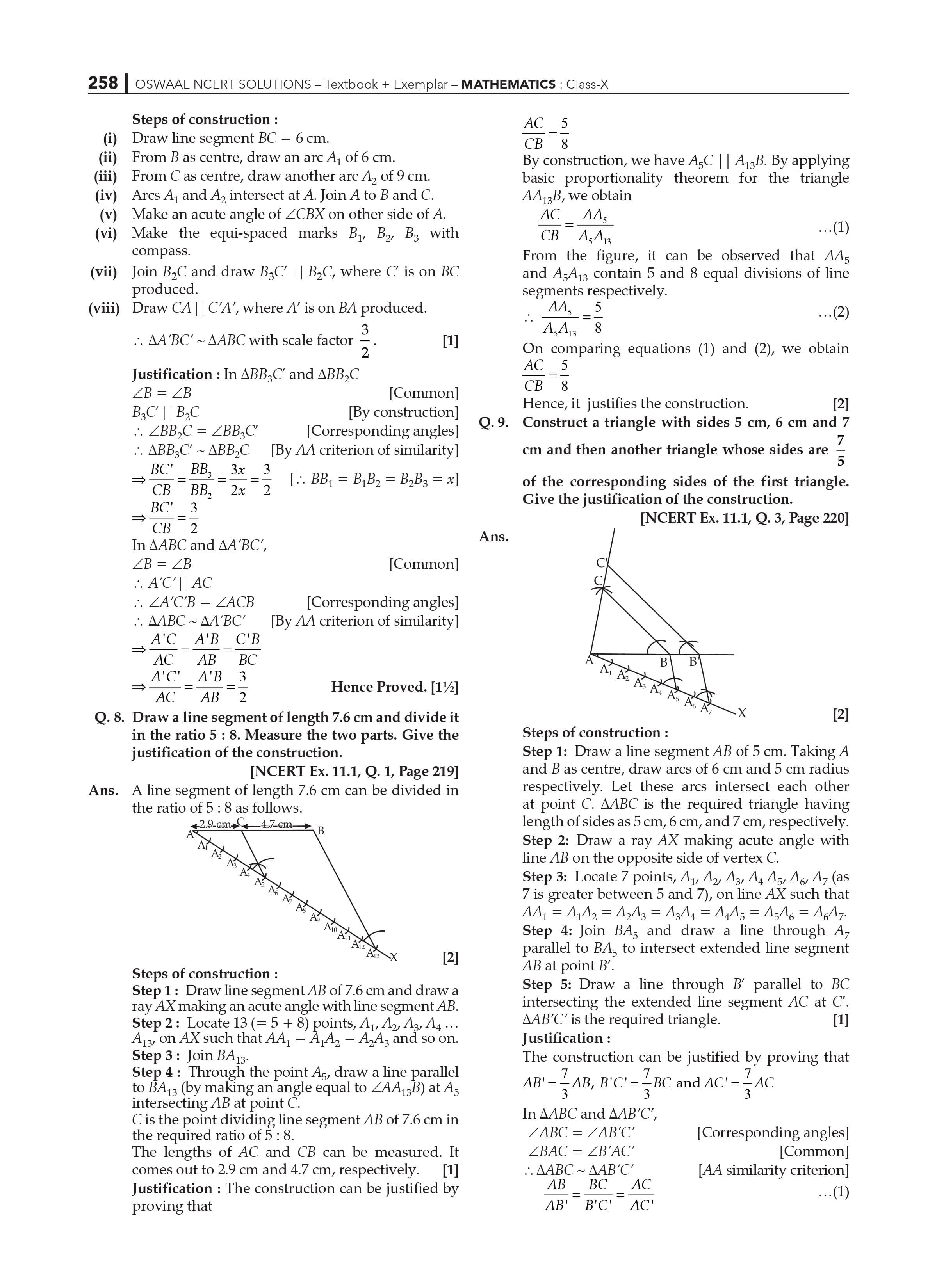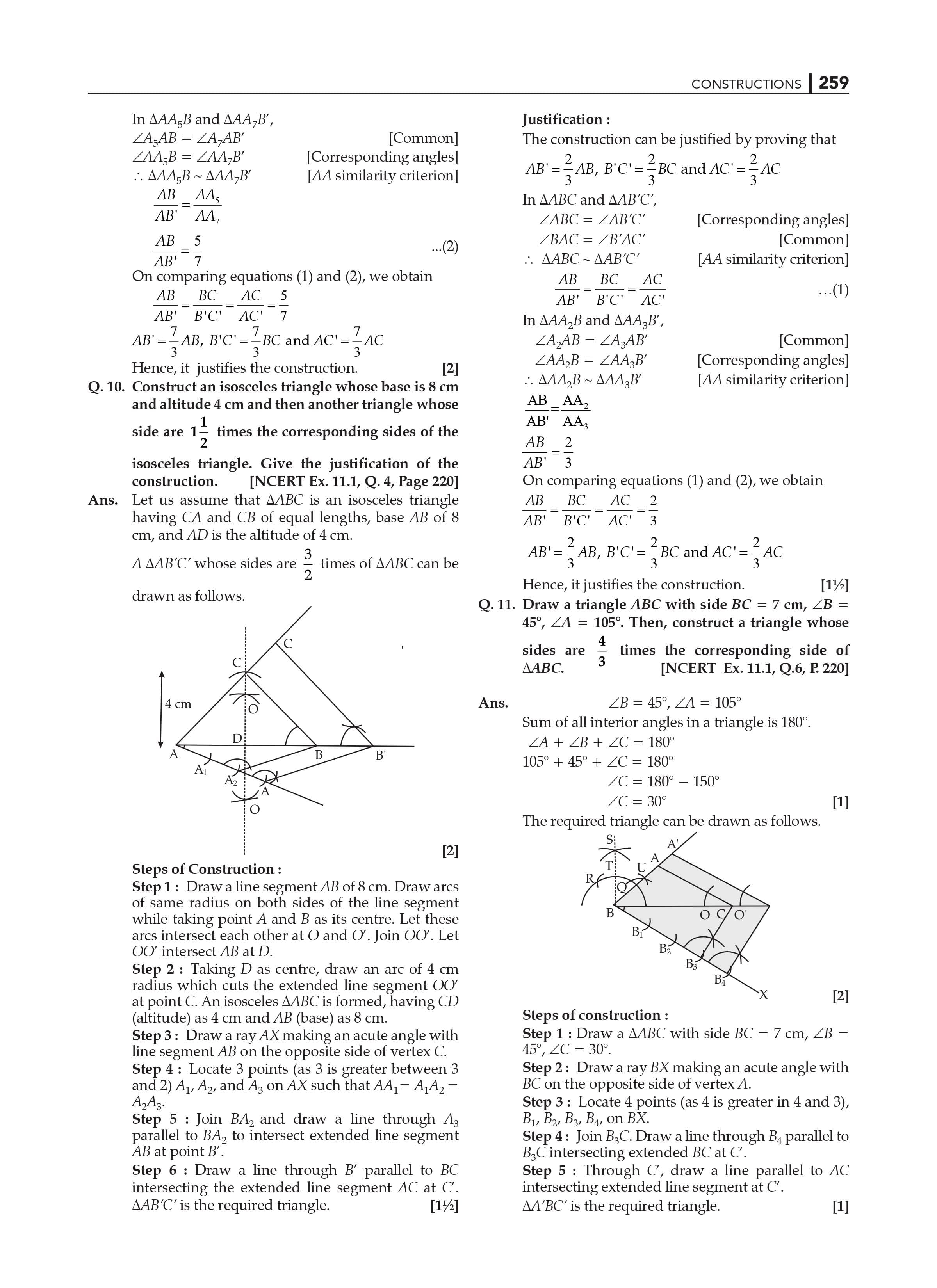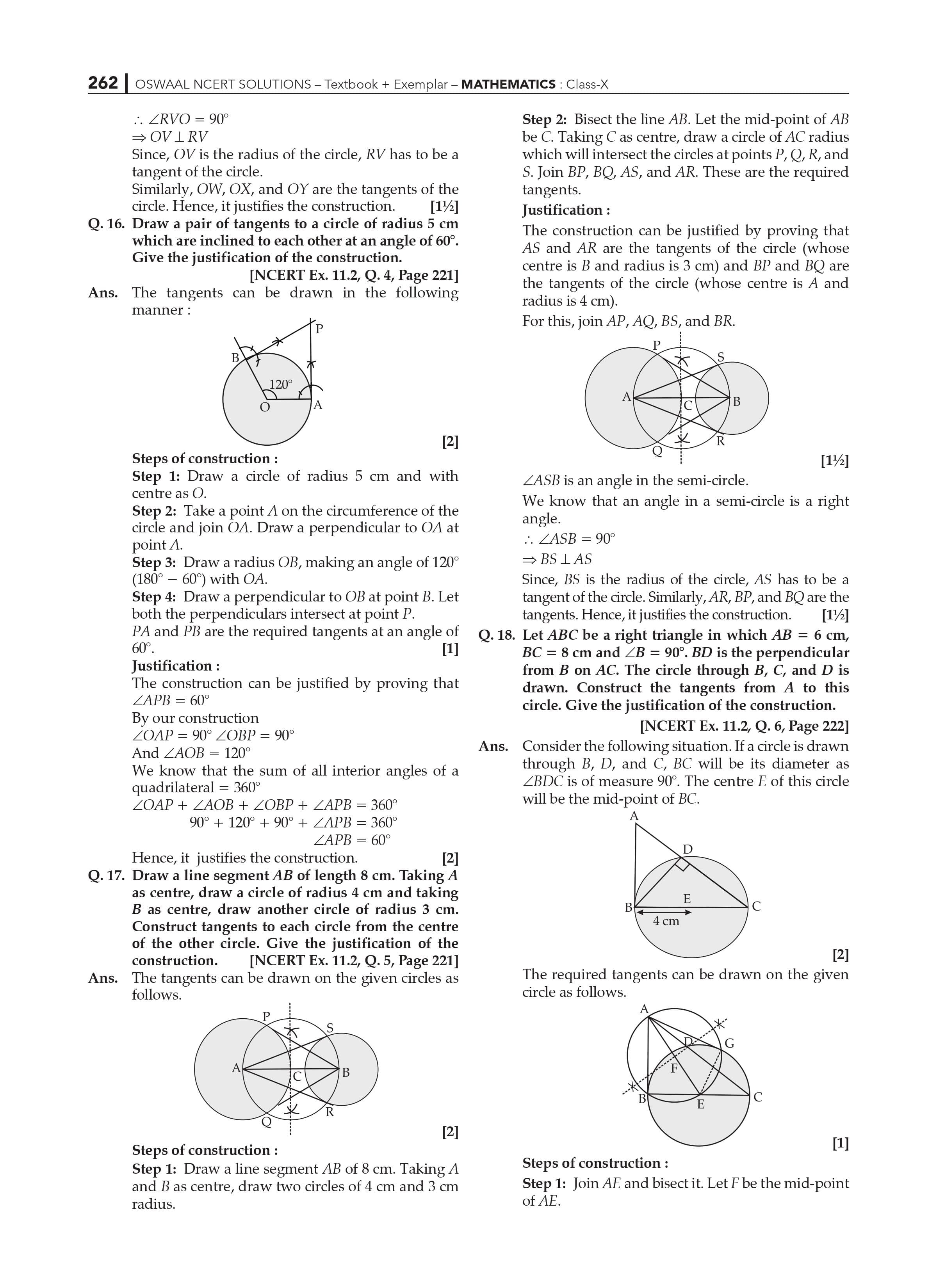The Class 10 Maths Chapter 11 Constructions of NCERT Solutions includes topics related to construction of line segments, division of line segments, construction of tangents to a circle etc. For these Constructions you’ll need to have a pre requisite knowledge of the topics covered in Class 9 such as drawing a line segment, how to bisect a line segment, construction of various triangles etc.

Chapter 11 Constructions is one of the parts of the geometry, and it has a total weightage of 15 marks in the 2023 examinations. This makes Construction a very scoring and important chapter for the exams. One question of 4 marks from this chapter is frequently asked in the CBSE 2023.

Construction of line segments, division of a line segment, construction of a circle, constructions of tangents to a circle by making use of analytical approach are some of the main topics that have been covered in Chapter 11 Constructions Maths NCERT Solutions for Class 10.

 Extra Information: NCERT Exemplar for Class 10 Maths Chapter 11 CBSE Notes for Class 10 Maths Chapter 11

Following are some of the concepts covered in Class 10 NCERT Chapter 11 Constructions of

Class 10 Maths NCERT Chapter 11 Constructions include major subjects as:

11.1 Introduction

11.2 Division of a Line Segment

11.3 Constructions of Tangents to a circle

11.4 Summary

Class 10 Maths Chapter 11 Constructions includes the two exercises having variety of questions:

This chapter will make use of the basics taught in class 9 such as the construction of a line segment, bisecting an angle, constructing a perpendicular bisector of a line segment, construction of triangles etc.

Practicing the NCERT Solutions for Class 10 might be highly important for the CBSE 2023 examinations.

Class 10 Maths NCERT Solutions of Chapter 11 Constructions Highlights:

NCERT Solutions assist students in solidifying their knowledge in constructing line segments, dividing line segments, tangents to Circles.

Diagrams are used to answer questions, making learning more dynamic and thorough.

The language used in NCERT Solutions is simple and easy to grasp.

Students benefit from a step-by-step approach to problem-solving.

Allows learners to work through challenging problems in their own time.

NCERT Solutions for various courses and disciplines are also available for students to use. Our well-experienced professors create these answers to offer clarity on essential ideas and problem-solving abilities.

Students can also obtain a firm grasp of critical topics by referring to additional study resources available at our website.

Along with the NCERT Solutions, college students also can exercise the alternative textbook answers to apprehend the ideas in a better way.

Frequently Asked Questions for Class 10 Maths Chapter 12 NCERT Solutions:

1. What are the benefits of studying NCERT Solutions for Class 10 Maths Chapter 11 Constructions?

Answer: NCERT Class 10 Maths Chapter 11 Constructions solutions assist students in obtaining a summary of the question paper format, which includes a range of questions, such as usually repeated questions, brief and long response type questions, multiple-choice questions, marks, and so on. The more problems you solve, the more confident you will be in your achievement.

1. Mention exam-relevant concepts in NCERT Solutions for Class 10 Maths Chapter 11 Constructions?

Answer: The most important exam topics in NCERT Solutions for Class 10 Maths Chapter 11 are construction of line segments, division of a line segment, construction of a circle, constructions of tangents to a circle and finally a summary of the entire chapter. Also make sure that your class 9 Constructions basic concepts are clear, only then you’ll be able to do Constructions in class 10.

1. What is the number of exercises in NCERT Solutions for Class 10 Maths Chapter 11 Constructions?

Answer:  Class 10 Maths Chapter 11 Constructions includes the two exercises having variety of questions: The Class 10 Maths Chapter 11 Constructions NCERT Solutions includes topics related to construction of line segments, division of line segments, construction of tangents to a circle etc.